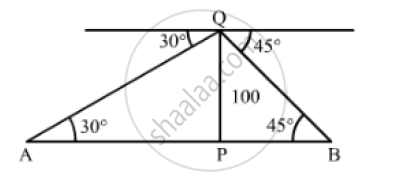# From the Point of a Tower 100m High, a Man Observe Two Cars on the Opposite Sides to the Tower with Angles of Depression 30° and 45 Respectively. Find the Distance Between the Cars - Mathematics

From the point of a tower 100m high, a man observe two cars on the opposite sides to the tower with angles of depression 30° and 45 respectively. Find the distance between the cars

#### SolutionLet PQ be the tower
We have,
PQ = 100m, ∠PQR = 30° and ∠PBQ = 45°
In ΔAPQ,

tan 30° = (PQ)/(AP)

⇒ 1/ sqrt(3) = 100/(AP)

⇒AP = 100 sqrt(3) m

Also, in ΔBPQ,

 tan 45° = (PQ)/(BP)

⇒ 1 = 100/(BP)

⇒ BP = 100M

Now , AB = AP+ BP

= 100 sqrt(3) + 100

= 100( sqrt(3) +1)

= 100 xx (1.73 +1 )

 = 100 xx 2.73

= 273 m

So, the distance between the cars is 273m.

Concept: Heights and Distances
Is there an error in this question or solution?
Chapter 14: Height and Distance - Exercises

#### APPEARS IN

RS Aggarwal Secondary School Class 10 Maths
Chapter 14 Height and Distance
Exercises | Q 13

Share